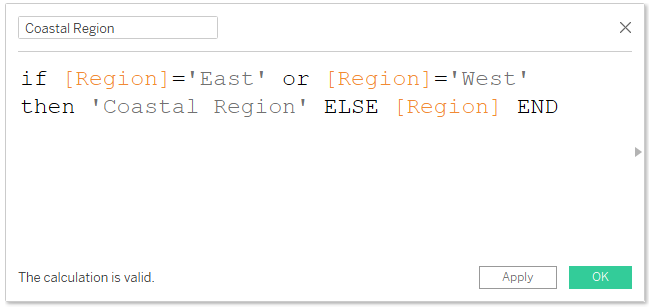top of page
Search
• Bernard K

# Tableau calculated field errorsCalculated fields allow you to create a new data from the data that already exist in the Tableau data source. And by creating a calculated field – you’re essentially creating a new field in the data source. While creating calculated fields, you may or will encounter several calculations errors – some of which we’re going to explore in this article.

Some of the common calculated field errors you will encounter include.

### Mixing aggregates and non-aggregates arguments

One of the common calculation errors in Tableau, occurs when you try mixing aggregate arguments with non-aggregate ones. For example, in this calculation am trying to compute Profit Ratio by mixing a non-aggregated argument (Profit) with aggregated argument (SUM(Sales)) ……Which returns the error message ‘Cannot mix aggregate with non-aggregate arguments with this function(This can be corrected by ensuring that you either use a combination of aggregate arguments or non-aggregate arguments – in this case, to compute Profit Ratio I need to aggregate both the arguments as shown below - SUM(Profit)/SUM(Sales)

### A missing closing parenthesis or comma

Another common calculated field error occurs when you leave a comma or the closing parenthesis in your calculation. For example, in this case am computing the ‘First Name’ of the Customer Name by separating the first part of the string using the space as the common separator. However, have left out the comma which returns the error message on missing closing parenthesis or comma.(This can be corrected by inserting a comma (,) between the specified common separator and the identifier – as show in the view above).

### Syntax error

Another common calculation error is the syntax error – commonly seen when something is missing in the calculation. For example, in this case am creating a new calculation - in which am going to combine the ‘East’ and ‘West’ region to create a new region called ‘Coastal Region’, while retaining the rest of regions in their default names. However, due to a missing argument (that’s I didn’t specify what the calculation should return if the Region is different from the declared region), the calculation returns a syntax error.This can be corrected by inserting the missing argument as shown below (whereby the calculation returns the default regions).### Mixing strings with floats/integers

Another common calculation error occurs when you mix strings with integers/floats. For example, in this case am dividing Order Id (a string) with Sales (a float) which returns the error message ‘Can’t divide string values by float value’.Same happens when you mix strings with floats using other mathematical operators such as addition, multiplication, subtraction etc. This can be corrected by ensuring that you don’t mix these data types in your arithmetic calculations.

### Unterminated string

This error appears when you fail to close an open string in a calculation. For example, in this case am combining the ‘East’ and ‘West’ regions to create a new region called ‘Coastal Region’. But because the string ‘Coastal Region’ is open within the calculation – I’ll get the error message ‘Unterminated stringThis can be corrected by ensuring that you close the open string.

### Failing to aggregate results in an LOD calculation

When you forget to aggregate the results of a level of detail (LOD) expression – you will get the error message ‘Results of a level of detail expression must be an aggregate’.This can be corrected by simply aggregating the results of the LOD expression.

More calculation errors can be identified by hovering/selecting on the calculated field section highlighted in red for details.